# Let's Get Started

Let's investigate real-world applications of graphs of quadratic functions.  You will analyze graphs of quadratic functions and interpret those graphs within the context of the situation.

TEKS Standards and Student Expectations

A(7) Quadratic functions and equations. The student applies the mathematical process standards when using graphs of quadratic functions and their related transformations to represent in multiple ways and determine, with and without technology, the solutions to equations. The student is expected to:

A(7)(A) graph quadratic functions on the coordinate plane and use the graph to identify key attributes, if possible, including x-intercept, y-intercept, zeros, maximum value, minimum values, vertex, and the equation of the axis of symmetry

Resource Objective(s)

Given the graph of a situation represented by a quadratic function, the student will analyze the graph and draw conclusions.

Essential Questions

What does the vertex of the graph represent?

How can you tell by looking at the graph if the "a" value is positive or negative?

Vocabulary

# Introduction

Quadratic functions are everywhere! Watch the following video to review important properties of quadratic functions and see several examples of real-world applications.

Source
Parabolas – A Quick Review, stelladuma, YouTube

We're going to investigate and analyze graphs of quadratic functions and then interpret those graphs within the context of the situation.

# Interpreting Direction and Width of Quadratic Functions

We're going to analyze the graphs of quadratic functions. First let's focus on the direction in which a parabola opens and the width of the parabola itself.This is exciting! A smile is forming on your face.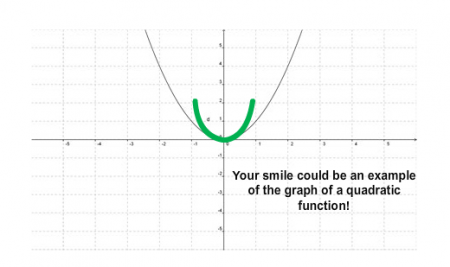The graph of a quadratic function is often referred to as a parabola with the equation y = ax2 + c. The coefficient, "a," describes the direction and width of the parabola, and the constant, "c," moves the parabola up and down.

Examine the picture below to see parabolas in a famous marketing symbol. Use the example to answer the questions that follow. Click on the question to check your answer.Source: McDonald's Golden Arches, The Consumerist, Flickr

Practice

The graphs below are all in the form y = ax². Use the graphs to answer the questions below.  To get a hint, click on the question.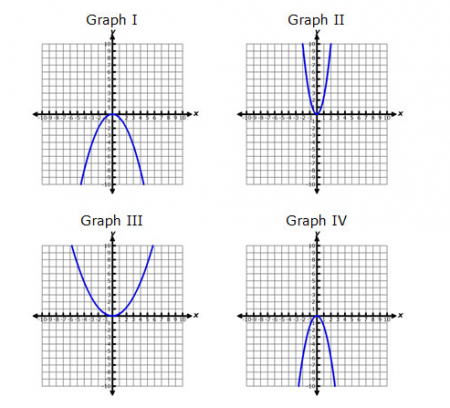A smirk, or a Mona Lisa style smile, appears to be wider than the parabola representing the parent function. This parabola has a value of "a" that is between 0 and 1.

# Interpreting Vertices of Quadratic Functions

Watch the video below. As you do so, notice the parabolas being created by the experimenters.

Source
Diet Coke + Mentos, zorro13, YouTube

This graph represents the height of a diver vs. the time after the diver jumps from a springboard. Answer the following questions based on the information.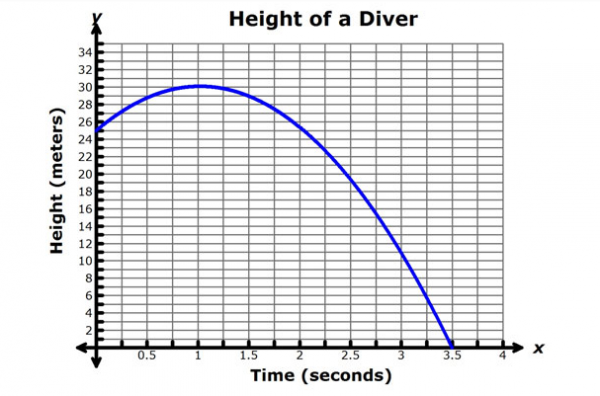# Interpreting Solutions of Quadratic Functions

The solutions of a quadratic function are the zeros. This is where the graph of the quadratic function crosses the x–axis.

Example

The Kemah Boardwalk has a new game that offers a \$1,000 bonus for the person with the highest score. Juane and Mirza decide to join the competition. A ball is put on the end of a lever that is on a table. Buckets are located at different distances from the lever. Each time you hit the lever, it launches a ball. If the ball lands in a bucket, you earn points depending on the distance the bucket is from the original starting point.

The object of the game is to earn the most points. Here are how a player earns points:

1. If the ball does not go into a bucket, 0 points are earned.
2. If the ball goes into the first bucket, 5 points are earned.
3. If the ball goes into the second bucket, 10 points are earned.
4. If the ball goes into the third bucket, 15 points are earned.
5. If the ball goes into the fourth bucket, 25 points are earned.

Here is how to play the game:

1. Press down on the lever to release the ball.
2. The ball goes (hopefully) into a bucket.
3. Each player tosses the ball three times for his or her total score.

Use the interactive activity to imitate the game that Mirza and Juan are playing. Toss the ball by clicking on the left end of the lever. Toss the ball three times for Mirza and then three times for Jose. Make sure to write down the points each competitor earned for each toss.

When Mirza and Juan were playing the bucket game, the number of points earned was based on where the parabola of the ball's path crossed the x-axis. There are actually two points of intersection--the first one is located at the origin, and the second one is located at the bucket.

If a quadratic function has its vertex on the x-axis, there is only one solution.

If a quadratic function does not cross the x-axis, there are no real number solutions.

Example

Bernadette throws the javelin for her school’s track and field team. The graph below shows the height and horizontal distance traveled by the javelin for three of Bernadette’s practice throws.

Use the graph to answer the following questions.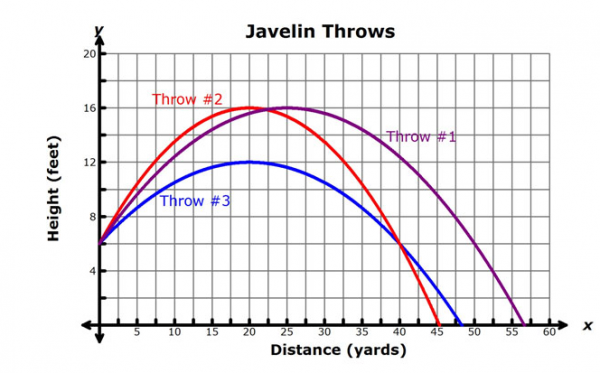# Summary

You've investigated real-world applications of graphs of quadratic functions. In particular, you explored ways to interpret the width and direction of a parabola, the vertex of a parabola, and solutions of a parabola.

The graph below shows important attributes of the graph of a parabola that you can use to analyze and interpret the graphs of quadratic functions.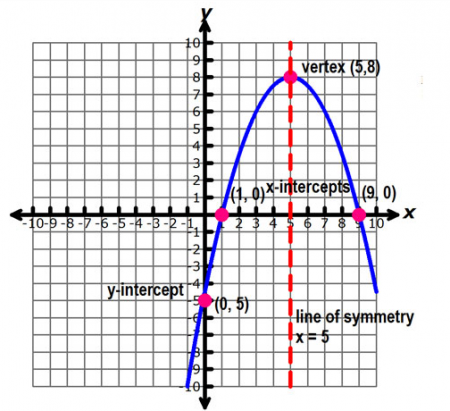The y-intercept is the point where the parabola crosses the y-axis.

The x-intercept is the point, or points, where the parabola crosses the x-axis. There may be 0, 1, or 2 x-intercepts, depending on the parabola.

The vertex is the turning point in a parabola. If the parabola opens downward, the vertex is a maximum point, and if the parabola opens upward, the vertex is a minimum point.

The line of symmetry is a vertical line passing through the vertex. A parabola is symmetric about this line, meaning that pairs of points in the parabola are equidistant from this line.